Courses
Courses for Kids
Free study material
Free LIVE classes
More

# Perpendicular Bisector and Angle Bisector TheoremLIVE
Join Vedantu’s FREE Mastercalss

## An Introduction to the Perpendicular Bisector and Angle Bisector Theorem

We come to encounter a variety of shapes in geometry. Among them is a triangle. To address issues involving triangles, we have various theorems at our disposal. are two examples. These crucial triangle theorems will be covered in this essay. A line known as a bisector divides an angle or a line into two parts of equal size. The midpoint of a segment is always contained in the segment's bisector. The perpendicular bisector theorem will be discussed first.

## What is a Perpendicular Bisector?

A line segment that crosses another line segment at a right angle and splits that another line into two equal portions at its middle is known as a perpendicular bisector.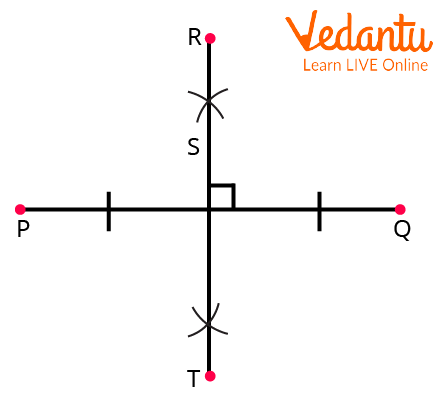Perpendicular Bisector

## What is the Perpendicular Bisector Theorem?

According to the perpendicular bisector theorem, every point on the perpendicular bisector is equally spaced from both of the line segment's endpoints.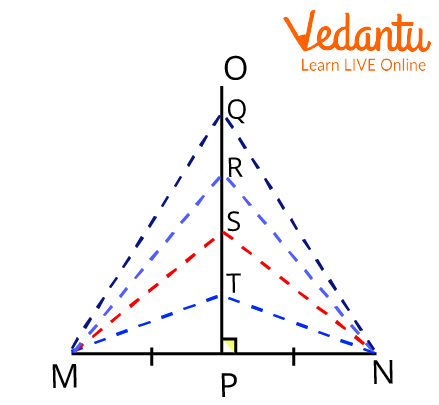Perpendicular Bisector Theorem

In the above figure,

$\begin{array}{l}MT\; = \;NT\;\;\\MS\; = \;NS\;\;\\MR\; = \;NR\;\;\\MQ\; = \;NQ\end{array}$

## Perpendicular Bisector Theorem Proof

Take a look at the diagram below, where C is just a random point on AB's perpendicular bisector (which intersects AB at D):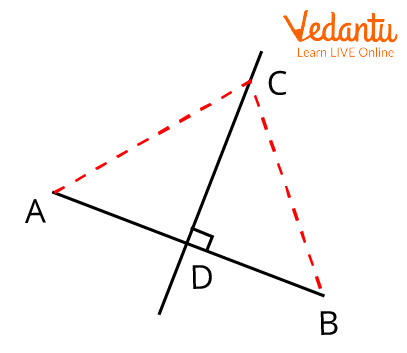Perpendicular Bisector Proof

Compare the $\triangle ACD$ and $\triangle BCD$.

$\begin{array}{l}AD = BD\\CD = CD(common)\\\angle ADC = \angle BDC = {90^ \circ }\end{array}$

By the SAS rule of congruence, the triangle ACD and triangle BCD are congruent.

Hence proved.

## What is Angle Bisector Theorem?

According to the Angle Bisector Theorem, the angle bisector of a triangle divides the other side in such a way that the ratio of the two line segments is equal to the ratio of the other two sides. Due to the angle bisector, the lengths of the other two triangle sides are equal to the relative lengths of the opposite side. All varieties of triangles can be analysed using the angle bisector theorem.

## Angle Bisector Theorem Statement

The angle bisector theorem states that a triangle's angle bisector separates its opposite side into two pieces that are proportional to its other two sides.

## Angle Bisector Theorem Proof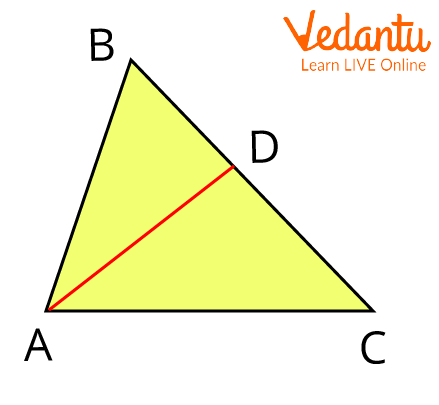Angle Bisector Theorem Proof

By utilising trigonometry in this case, we can quickly demonstrate the angle bisector theorem. Using the law of sines, we can write the following in the triangles ABD and ACD (in the preceding diagram);

$\begin{array}{l}\dfrac{{|AB\lvert}}{{|BD\lvert}}\; = \dfrac{{\;\sin \angle BDA}}{{\sin \angle BAD}}\;\;\; \ldots \left( 1 \right)\\\dfrac{{|AC|}}{{|DC|}}\; = \dfrac{{\;\sin \angle ADC}}{{\sin \angle DAC}}\;\;\; \ldots \left( 2 \right)\end{array}$

Angles ∠ ADC and ∠ BDA are referred to as adjacent supplementary angles since they form a linear pair.

Due to the sine of supplementary angles being equal,

$\sin \;\angle \;BDA\; = \;\sin \;\angle \;ADC\;\;\;\;\; \ldots ..\left( 3 \right)$

Also,

$\angle \;DAC\; = \;\angle \;BAD\;$(Since AD is an angle bisector)

Therefore,

$\sin \;\angle \;BDA\; = \;\sin \angle \;ADC\;\;\;\; \ldots \left( 4 \right)$

Since the RHS of equations 1 and 2 are equal, it follows that the LHS will likewise be equally based on equations 3 and 4.

$\dfrac{{\left| {BD} \right|}}{{\left| {DC} \right|}} = \dfrac{{\left| {AB} \right|}}{{\left| {AC} \right|}}$

Hence Proved.

## Applications of the Perpendicular Bisector and Angle Bisector Theorem

• A triangle's lengths can also be determined using the perpendicular bisector theorem in combination with other theorems.

• Due to the fact that an angle bisector divides the side opposite the angle into two segments proportional to the triangle's other two sides, the Angle Bisector Theorem aids in determining the lengths of sides of triangles that are unknown.

## Interesting Fact

Both angle bisector and perpendicular bisector theorems' converses are true as well.

## Solved Examples of the Perpendicular Bisector and Angle Bisector Theorem

1. The perpendicular bisector of triangle ABC on line segment BC in a pyramid is line segment AD. Find the length of side AC if AB is 20 feet long and BD is 7 feet long.

Ans: It is assumed that AD is the line segment's perpendicular bisector on BC.

Therefore, any point on line segment AD is equally far from points B and C according to the perpendicular bisector theorem. Meaning that AB = AC.

Therefore,

$AC\; = \;20\;feet$

2. Can we state that the vertex between equal sides in any equilateral or isosceles triangle lies on the perpendicular bisector of the base?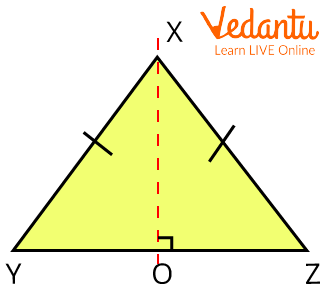Triangle XYZ

Ans: From vertex X, create a perpendicular that intersects segment YZ at point O.

The inverse of the perpendicular bisector theorem is used to demonstrate that

$OY = OZ$

If $XY\; = \;XZ$.

On the perpendicular bisector of the triangle's base, YZ is where vertex X is located.

3. Using the angle bisector theorem, determine the value of x for the given triangle.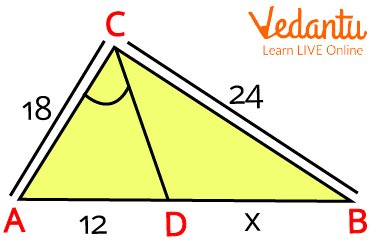Triangle ABC

Ans: Given data,

$AD=12$, $AC=18$, $BC=24$, and $DB=X$.

By the angle bisector theorem,

$\dfrac{{AD\;}}{{AC}} = \dfrac{{\;DB}}{{BC}}$

Now replace the values, we get

$\begin{array}{l}\dfrac{{12}}{{18}} = \dfrac{{\;X\;}}{{24}}\\X = \dfrac{{\;2\;}}{3}\left( {24} \right)\\X = \;2\left( 8 \right)\;\\X = \;16\end{array}$

Therefore, the value of X is 16.

## Important Points to Remember

• The SAS congruence criterion can be used to demonstrate the perpendicular bisector theorem and its opposite.

• In order to create something in the middle and an equal distance from each endpoint, the perpendicular bisector theorem is employed in the construction of buildings, bridges, and other structures.

## Important Formulas from the Theorem

• According to the perpendicular bisector theorem, if a bisector CD bisects the triangle ABC in two triangles, then

$\angle ADC = \angle BDC = {90^ \circ }$

• According to the angle bisector theorem, for a triangle ABC having a bisector AD

$\dfrac{{\left| {BD} \right|}}{{\left| {DC} \right|}} = \dfrac{{\left| {AB} \right|}}{{\left| {AC} \right|}}$

## Conclusion

The perpendicular bisector and angle bisector theorems and their explanations have been covered in-depth in this article. We may infer from the examination of these two theorems above that each of them is important to Mathematics and has a variety of implications. The theorems hold valid similarly.

Last updated date: 30th Sep 2023
Total views: 104.1k
Views today: 2.04k

## FAQs on Perpendicular Bisector and Angle Bisector Theorem

1. What does the Angle Bisector Theorem's converse say?

According to the converse of the angle bisector theorem, the point on the opposite side of an angle must be on its angle bisector if the sides of a triangle satisfy the following condition: "If a line drawn from a vertex of a triangle divides the opposite side into two parts such that they are proportional to the other two sides of the triangle." Given that the sides are in proportion, in this case, we can infer that the line, ray, or segment in question is the angle's bisector.

2. What is the converse of the perpendicular bisector theorem?

The perpendicular of a line segment is the line segment that divides another line segment into two halves across the midpoint at 90o. According to the opposite of the perpendicular bisector theorem, a point is on the perpendicular bisector of a line segment if it is equally spaced from both of the endpoints of the line segment in the same plane. The perpendicular bisector theorem, when combined with other theorems, can be used to find the lengths of a triangle.

3. What does angle bisector imply and what are its properties?

A ray, segment, or line that divides a given angle into two equal angles is known as an angle bisector. When something is bisected or bisected, it is split into two equal sections. In geometry, an angle bisector is a line or ray that divides a triangle into two equal angles.

Basic Properties:

• An angle is divided into two equal pieces by an angle bisector.

• Any point on an angle's bisector is equally spaced from the angle's sides or arms.

• It divides the opposite side of a triangle into the product of the lengths of the other two sides.